International
Tables for
Crystallography
Volume B
Reciprocal space
Edited by U. Shmueli

International Tables for Crystallography (2006). Vol. B, ch. 5.2, p. 555   | 1 | 2 |

## Section 5.2.12. Multislice

A. F. Moodie,a J. M. Cowleyb and P. Goodmanc

aDepartment of Applied Physics, Royal Melbourne Institute of Technology, 124 La Trobe Street, Melbourne, Victoria 3000, Australia,bArizona State University, Box 871504, Department of Physics and Astronomy, Tempe, AZ 85287-1504, USA, and cSchool of Physics, University of Melbourne, Parkville, Australia 3052

### 5.2.12. Multislice

| top | pdf |

Multislice derives from a formulation that generates a solution in the form of a Born series (Cowley & Moodie, 1962). The crystal is treated as a series of scattering planes on to which the potential from the slice between z and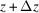is projected, separated by vacuum gaps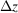, not necessarily corresponding to any planes or spacings of the material structure. The phase change in the electron beam produced by passage through a slice is given by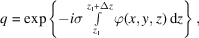and the phase distribution in the x, y plane resulting from propagation between slices is given by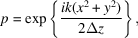where the wavefront has been approximated by a paraboloid. Thus, the wavefunction for the (n + 1)th slice is given by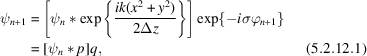whereis the convolution operator (Cowley, 1981).

This equation can be regarded as the finite difference form of the Schrödinger equation derived by Feynman's (1948) method. The calculation need be correct only to first order in. Writing the convolution in equation (5.2.12.1)explicitly, and expanding in a Taylor series, the integrals can be evaluated to yield equation (5.2.3.1)(Goodman & Moodie, 1974).

If equation (5.2.12.1)is Fourier transformed with respect to x and y, the resulting recurrence relation is of the form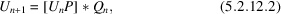where P and Q are obtained by Fourier transforming p and q above. This form is convenient for numerical work since, for a perfect crystal, it is: discrete, as distinct from equation (5.2.12.1)which is continuous in the variables [see IT C (2004, Section 4.3.6.1)]; numerically stable at least up to 5000 beams; fast; and only requires a computer memory proportional to the number of beams (Goodman & Moodie, 1974).

### References

International Tables for Crystallography (2004). Vol. C. Mathematical, physical and chemical tables, edited by E. Prince. Dordrecht: Kluwer Academic Publishers.Google Scholar
Cowley, J. M. (1981). Diffraction physics, pp. 26–30. Amsterdam: North-Holland.Google Scholar
Cowley, J. M. & Moodie, A. F. (1962). The scattering of electrons by thin crystals. J. Phys. Soc. Jpn, 17, Suppl. B11, 86–91.Google Scholar
Feynman, R. (1948). Space–time approach to non-relativistic quantum mechanics. Rev. Mod. Phys. 201, 367–387.Google Scholar
Goodman, P. & Moodie, A. F. (1974). Numerical evaluation of N-beam wave functions in electron scattering by the multislice method. Acta Cryst. A30, 280–290.Google Scholar